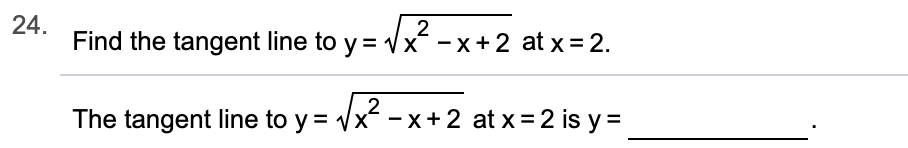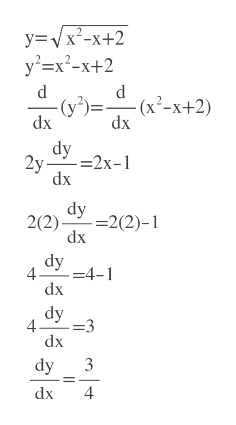# 24Find the tangent line to y2x^ -x+ 2 at x=2.2The tangent line to y x-x + 2 at x = 2 is y=

Question
1 views

Can you help me step by step?help_outlineImage Transcriptionclose24 Find the tangent line to y 2 x^ -x+ 2 at x=2. 2 The tangent line to y x-x + 2 at x = 2 is y= fullscreen
check_circle

Step 1

We first have to find y at x=2

y=2

Step 2

We need slope dy/dx at x=2 and y=2

First square both sides of the given expression

then differentiat...help_outlineImage Transcriptionclosey=/x2-x+2 y2x2-+2 d (y2) dx dx - (x2-x+2) dy 2у -2х-1 dx dy =2(2)-1 2(2) dx dy 4 4-1 dx dy 4 =3 dx dy 3 4 dx fullscreen

### Want to see the full answer?

See Solution

#### Want to see this answer and more?

Solutions are written by subject experts who are available 24/7. Questions are typically answered within 1 hour.*

See Solution
*Response times may vary by subject and question.
Tagged in

### Other